# Information, transmission rate of

(diff) ← Older revision | Latest revision (diff) | Newer revision → (diff)

A quantity characterizing the amount of information that is contained in the output signal of a communication channel relative to the input signal, calculated in a unit of time (cf. Information, amount of). Ifare stochastic processes in discrete or continuous time, being the input and output signals of a communication channel, then the quantity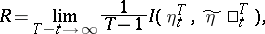(*)

is the transmission rate of information (if the limit exists). Here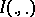is the amount of information,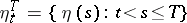is the segment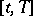ofandis analogously defined. The existence of the limit in (*) has been proved for the large class of channels in which the signalsand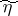are stationary and stationarily-related stochastic processes. An explicit computation of the transmission rate of information is possible, in particular, for a memoryless channel and a Gaussian channel. E.g., for a Gaussian channel, whose signalsandare Gaussian stationary processes forming a joint Gaussian stationary pair of processes, the transmission rate of information is given bywhere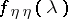and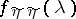are the spectral densities ofand, respectively, and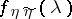is their joint spectral density.

How to Cite This Entry:
Information, transmission rate of. Encyclopedia of Mathematics. URL: http://encyclopediaofmath.org/index.php?title=Information,_transmission_rate_of&oldid=13228
This article was adapted from an original article by R.L. DobrushinV.V. Prelov (originator), which appeared in Encyclopedia of Mathematics - ISBN 1402006098. See original article